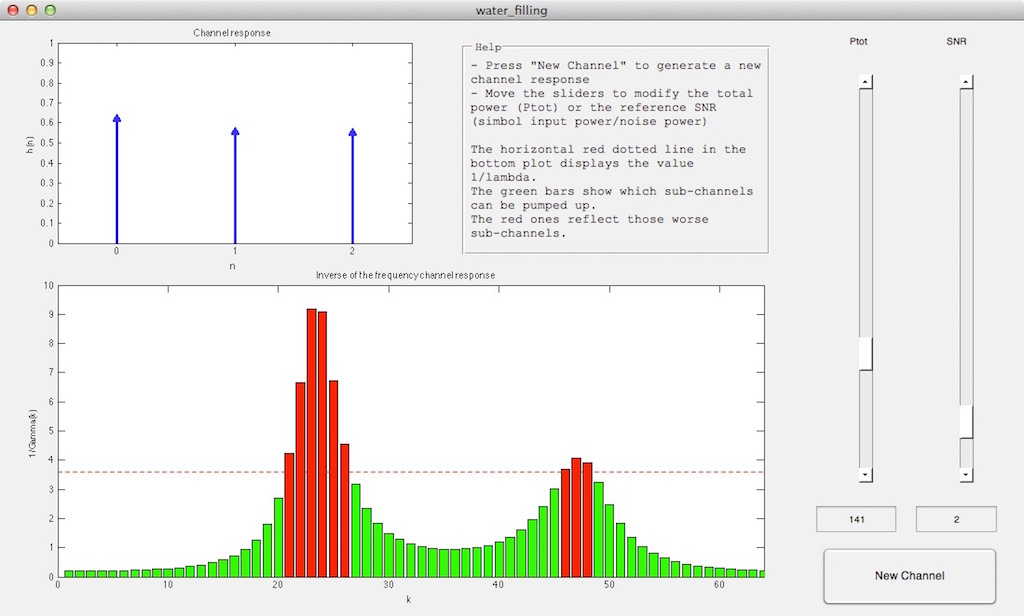### Water-filling algorithm for finding the optimal power allocation of each subchannel in OFDM systems

#### Introduction

The water-filling (also known as water pouring) algorithm allocates more (or less) bits and power to some subcarriers with larger (or smaller) SNR for maximizing the channel capacity. This implies that the water-filling algorithm requires a full knowledge of channel state information for each subcarrier on the transmitter side.

#### Code

This is a MATLAB GUI that allows one to compute and graphically represent the optimal power allocation for each band of an OFDM system using the water-filling algorithm.

For more details take a look at the help section in the tool.MATLAB GUI